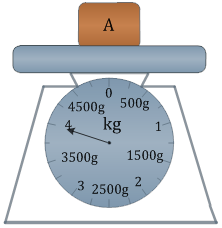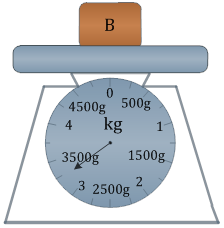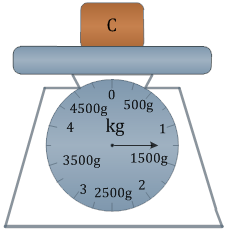### Sample Problem(a) A weighs kg g.

(b) B weighs kg g.

(c) C weighs kg g.

(d) is the heaviest. (Type “A”, “B”, or “C”).

(e) is the lightest. (Type “A”, “B”, or “C”).

(f) A is kg g heavier than C.

(g) A is kg g heavier than B.

(h) C is kg g lighter than B.

#### Solution

(a) A weighs 4 kg.

(b) B weighs 3 kg 250 g.

(c) C weighs 1 kg 250 g

(d) A is the heaviest.

(e) C is the lightest.

(f) 4 kg – 1 kg 250 g = 2 kg 750 g

(g) 4 kg – 3 kg 250 g = 750 g

(h) 3 kg 250 g – 1 kg 250 g = 2 kg